# How to Plot Mean and Standard Deviation in Pandas?

## How to Plot Mean and Standard Deviation in Pandas?

Errorbar is the plotted chart that refers to the errors contained in the data frame, which shows the confidence & precision in a set of measurements or calculated values. Error bars help in showing the actual and exact missing parts as well as visually display the errors in different areas in the data frame. Error bars are the descriptive behavior that holds information about the variances in data as well as advice to make proper changes to build data more insightful and impactful for the users.

Here we discuss how we plot errorbar with mean and standard deviation after grouping up the data frame with certain applied conditions such that errors become more truthful to make necessary for obtaining the best results and visualizations.

Modules Needed:

```pip install numpy
pip install pandas
pip install matplotlib```

Here is the DataFrame from which we illustrate the errorbars with mean and std:

 `# Import the necessary libraries to read` `# dataset and work on that` `import` `pandas as pd` `import` `numpy as np` `import` `matplotlib.pyplot as plt` `# Make the dataframe for evaluation on Errorbars` `df ``=` `pd.DataFrame({` `    ``'insert'``: [``0.0``, ``0.1``, ``0.3``, ``0.5``, ``1.0``],` `    ``'mean'``: [``0.009905``, ``0.45019``, ``0.376818``, ``0.801856``, ``0.643859``],` `    ``'quality'``: [``'good'``, ``'good'``, ``'poor'``, ``'good'``, ``'poor'``],` `    ``'std'``: [``0.003662``, ``0.281895``, ``0.306806``, ``0.243288``, ``0.322378``]})` `print``(df)`

Output: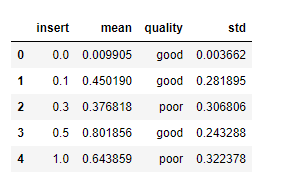Sample DataFrame

groupby the subplots with mean and std to get error bars:

 `# Subplots as having two types of quality` `fig, ax ``=` `plt.subplots()` `for` `key, group ``in` `df.groupby(``'quality'``):` `    ``group.plot(``'insert'``, ``'mean'``, yerr``=``'std'``,` `               ``label``=``key, ax``=``ax)` `plt.show()`

Output: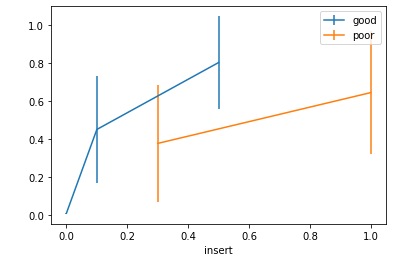Example 1: ErrorBar with group-plot

Now we see error bars using NumPy keywords of mean and std:

 `# Groupby the quality column using aggregate` `# value of mean and std` `qual ``=` `df.groupby(``"quality"``).agg([np.mean, np.std])` `qual ``=` `qual[``'insert'``]` `qual.plot(kind ``=` `"barh"``, y ``=` `"mean"``, legend ``=` `False``,` `          ``xerr ``=` `"std"``, title ``=` `"Quality"``, color``=``'green'``)`

Output: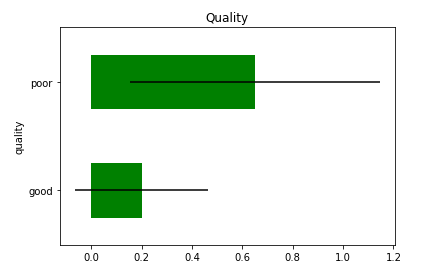Example 2: ErrorBar with Bar Plot

By the above example, we can see that errors in poor quality are higher than good instead of more good values in the data frame.

Now, we move with another example with data frame below: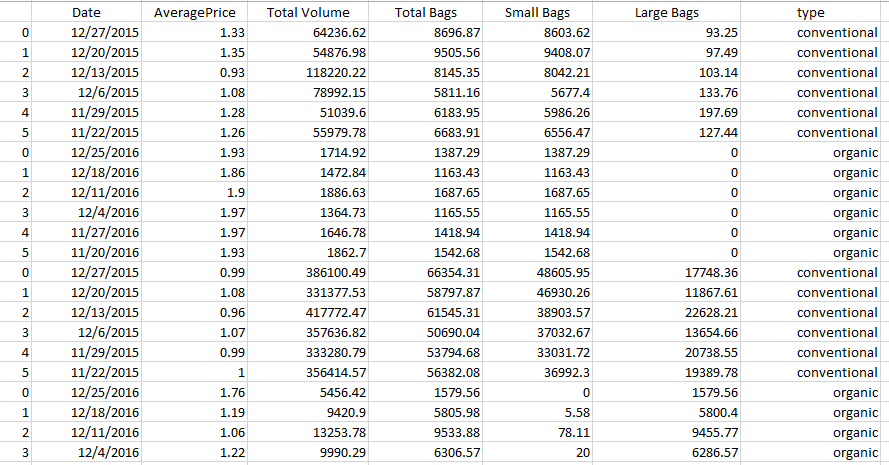Dataset – Toast

By the above data frame, we have to manipulate this data frame to get the errorbars by using the ‘type’ column having different prices of the bags. To manipulation and perform calculations, we have to use a df.groupby function that has a prototype to check the field and execute the function to evaluate result.

We are using two inbuilt functions of mean and std:

`df.groupby("col_to_group_by").agg([func_1, func_2, func_3, .....])`
 `# reading the dataset` `df ``=` `pd.read_csv(``'Toast.csv'``)` `df_prices ``=` `df.groupby(``"type"``).agg([np.mean, np.std])`

As we have to evaluate the average price, so apply this groupby on ‘AveragePrice’. Also, check the result of prices and with the visualization display the errorbars

 `prices ``=` `df_prices[``'AveragePrice'``]` `# checking for results` `prices.head()`

Output: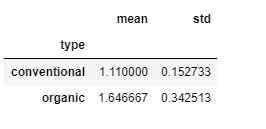Result: the aggregate value of  groupby()

Errorbar using Mean:

 `prices.plot(kind ``=` `"barh"``, y ``=` `"mean"``, legend ``=` `False``,` `            ``title ``=` `"Average Prices"``)`

Output: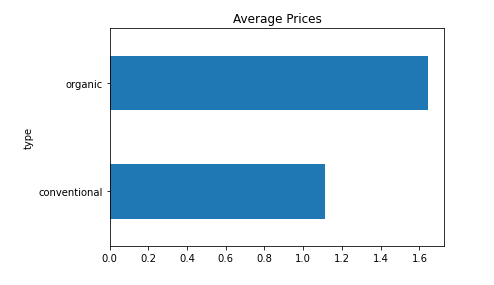Example 3: Errorbar with Mean

By the above visualization, it’s clear that organic has a higher mean price than conventional.

Errorbar using Standard Deviation (std):

 `prices.plot(kind ``=` `"barh"``, y ``=` `"mean"``, legend ``=` `False``,` `            ``title ``=` `"Average Prices"``, xerr ``=` `"std"``)`

Output: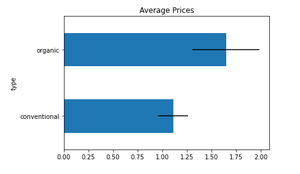Example 4: Errorbar with Std

• Errorbars are more obstacles.
• They are easy to execute with good estimation values.
• Relatively uniform because of complex interpretation power with a data frame.

Last Updated on March 1, 2022 by admin

## Python program to get all unique combinations of two ListsPython program to get all unique combinations of two Lists

Python program to get all unique combinations of two Lists The combination is a mathematical

## Interquartile Range and Quartile Deviation using NumPy and SciPyInterquartile Range and Quartile Deviation using NumPy and SciPy

Interquartile Range and Quartile Deviation using NumPy and SciPy Quartiles : A quartile is a

## Python Dictionary ComprehensionPython Dictionary Comprehension

Python Dictionary Comprehension Like List Comprehension, Python allows dictionary comprehensions. We can create dictionaries using simple

## Inner Class in PythonInner Class in Python

Inner Class in Python A Python is an Object-Oriented Programming Language, everything in python is related to

## Create a Snake-Game using Turtle in PythonCreate a Snake-Game using Turtle in Python

Create a Snake-Game using Turtle in Python A snake game is an arcade maze game which has

## get_attribute() element method – Selenium Pythonget_attribute() element method – Selenium Python

get_attribute() element method – Selenium Python get_attribute() element method – Selenium Python Difficulty Level : Basic

## Introduction to MatplotlibIntroduction to Matplotlib

Python | Introduction to Matplotlib Matplotlib is an amazing visualization library in Python for 2D

## Python – Itertools.chain()Python – Itertools.chain()

Python – Itertools.chain() The itertools is a module in Python having a collection of functions that are